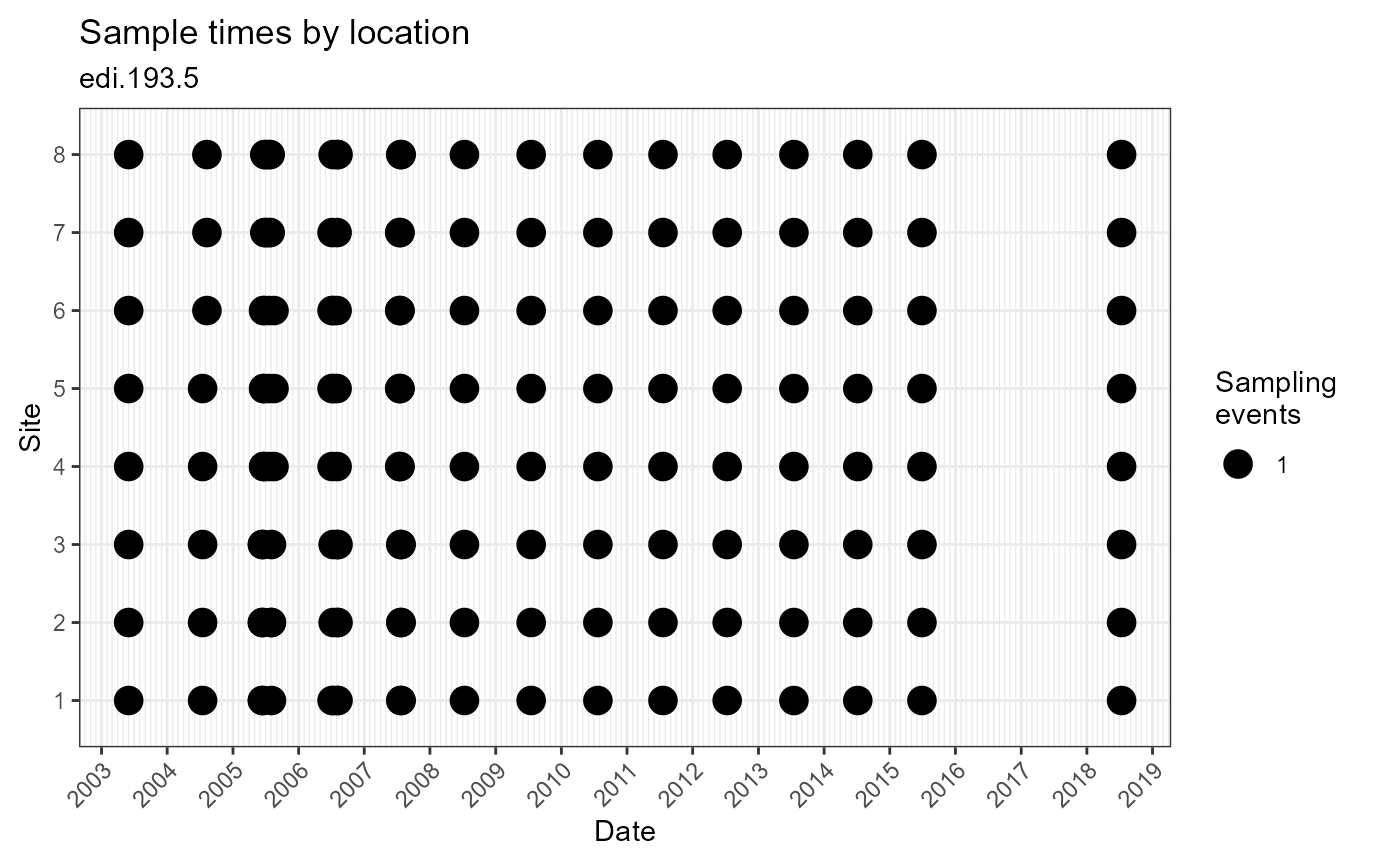Plot dates and times samples were collected or observations were made

plot_sample_space_time(data, id = NA_character_, alpha = 1, observation = NULL)

Arguments

data (list or tbl_df, tbl, data.frame) The dataset object returned by read_data(), a named list of tables containing the observation table, or a flat table containing columns of the observation table. (character) Identifier of dataset to be used in plot subtitles. Is automatically assigned when data is a dataset object containing the id field, or is a table containing the package_id column. (numeric) Alpha-transparency scale of data points. Useful when many data points overlap. Allowed values are between 0 and 1, where 1 is 100% opaque. Default is 1. (tbl_df, tbl, data.frame) DEPRECATED: Use data instead.

Value

(gg, ggplot) A gg, ggplot object if assigned to a variable, otherwise a plot to your active graphics device

Details

The data parameter accepts a range of input types but ultimately requires the 9 columns of the observation table.

Examples

if (FALSE) { # Read a dataset of interest dataset <- read_data("edi.193.5") # Plot the dataset plot_sample_space_time(dataset) # Flatten the dataset, manipulate, then plot dataset %>% flatten_data() %>% dplyr::filter(lubridate::as_date(datetime) > "2003-07-01") %>% dplyr::filter(as.numeric(location_id) > 4) %>% plot_sample_space_time() } # Plot the example dataset plot_sample_space_time(ants_L1)